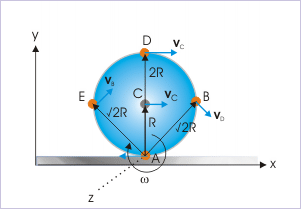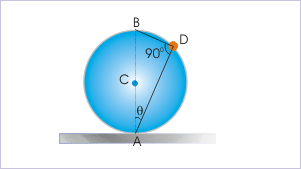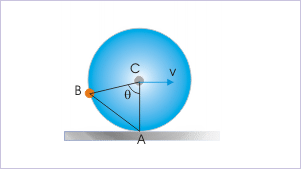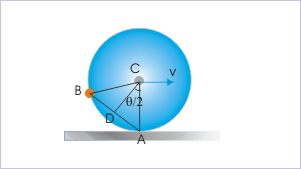19.2 Rolling as pure rotation  (Page 2/2)

 Page 2 / 2The linear velocity is tangential to the circular path of the particle about the instantaneous axis.

We must note that all velocity vectors are drawn perpendicular to position vectors, which are drawn from the point of contact "A". The magnitude of the velocity is given by :

$\begin{array}{l}v=\omega r\mathrm{sin}{90}^{0}=\omega r\end{array}$

where "r" is the linear distance of the position from the point of contact and "ω" is the angular velocity about the new axis through the point of contact or the axis through center of mass.

We can validate the assumption of rolling as pure rotation by calculating velocities of some of the positions (A,B,C,D and E) on the rim of the rotating disk.

$\begin{array}{l}{v}_{A}=\omega r=\omega x0=0\\ {v}_{B}=\omega r=\omega x\surd 2R=\surd 2{v}_{C}\\ {v}_{C}=\omega r=\omega xR={v}_{C}\\ {v}_{D}=\omega r=\omega x2R=2{v}_{C}\\ {v}_{E}=\omega r=\omega x\surd 2R=\surd 2{v}_{C}\end{array}$

These results are same as obtained by considering pure rolling motion equivalent to the combination of pure rotation and pure translation. As such, the equivalence of pure rolling as pure rotation about an axis through the point of contact and perpendicular to the plane of rotating disk is indeed true. It is also evident from the calculation details that this approach of analyzing rolling motion is simpler than the earlier approach as far as calculation of linear velocities of different positions within the rotating disk is concerned.

For calculating velocities of the positions on the rim of the rotating disk, there is a convenient trigonometric relation that can be used with ease. We consider a position on the rim of the disk making an angle θ with the vertical at the point of contact. The base of the triangle AB is the diameter of the circle. As such the angle ADB is a right angle (angle subtended by diameter on the circumference is right angle).The ring rolls with constant velocity.

$\begin{array}{l}\mathrm{cos}\theta =\frac{\mathrm{AD}}{\mathrm{AB}}=\frac{\mathrm{AD}}{\mathrm{2R}}\\ ⇒\mathrm{AD}=\mathrm{2R}\mathrm{cos}\theta \end{array}$

The linear velocity of the particle at the rim of the rotating disk, therefore, is :

$\begin{array}{l}⇒v=\omega r=\omega \mathrm{2R}\mathrm{cos}\theta =2\omega R\mathrm{cos}\theta \end{array}$

$\begin{array}{l}⇒v=2{v}_{C}\mathrm{cos}\theta \end{array}$

Problem : A ring rolls on a horizontal plane with a constant velocity, "v". Find the speed of a particle on the ring, making an angle "θ" with the center as shown in the figure.The ring rolls with constant velocity.

Solution : We can not apply the formula as derived earlier directly. Here, the given angle is at the center of the ring and not at the point of contact as needed to use the formula directly. Now, the linear velocity of the particle is given as :

$\begin{array}{l}{v}_{B}=\omega r\end{array}$

In order to find "r" i.e. "AB", we drop a perpendicular from the center of the circle.The ring rolls with constant velocity.

In the right angle ACD, we have :

$\begin{array}{l}\mathrm{AD}=R\mathrm{sin}\left(\frac{\theta }{2}\right)\\ ⇒\mathrm{AB}=2\mathrm{AD}=2R\mathrm{sin}\left(\frac{\theta }{2}\right)\end{array}$

Putting this expression for "r" in the equation :

$\begin{array}{l}{v}_{B}=\omega \mathrm{AB}=\omega 2R\mathrm{sin}\left(\frac{\theta }{2}\right)=2\omega R\mathrm{sin}\left(\frac{\theta }{2}\right)\end{array}$

But, we know that "ωR" equals speed of the center of mass.

$\begin{array}{l}{v}_{B}=2{v}_{C}\mathrm{sin}\left(\frac{\theta }{2}\right)\end{array}$

As given in the question, the speed of the center of mass is "v". Hence,

$\begin{array}{l}{v}_{B}=2v\mathrm{sin}\left(\frac{\theta }{2}\right)\end{array}$

Kinetic energy of rolling disk

We can determine kinetic energy of the rolling disk, considering it to be the pure rotation about an axis passing through point of contact and plane of disk. Mathematically,

$\begin{array}{l}K={K}_{R}=\frac{1}{2}{I}_{A}{\omega }^{2}\end{array}$

where " ${I}_{A}$ " is the moment of inertia of the disk about new axis. Now, using theorem of parallel axes, we have :

$\begin{array}{l}{I}_{A}={I}_{C}+M{R}^{2}\end{array}$

where "I" is moment of inertia about an axis passing through center of mass and perpendicular to the plane of rotating disk. Putting in the equation of kinetic energy, we have :

$\begin{array}{l}K=\frac{1}{2}{I}_{A}{\omega }^{2}\end{array}$

$\begin{array}{l}⇒K=\frac{1}{2}\left({I}_{C}+M{R}^{2}\right){\omega }^{2}\\ ⇒K=\frac{1}{2}{I}_{C}{\omega }^{2}+\frac{1}{2}M{R}^{2}{\omega }^{2}\\ ⇒K=\frac{1}{2}{I}_{C}{\omega }^{2}+\frac{1}{2}M{{v}_{C}}^{2}\\ ⇒K={K}_{R}+{K}_{T}\end{array}$

This result is same as that obtained by considering rolling as combined motion of pure rotation and pure translation. It again emphasizes the correctness of the original assumption that rolling can be treated as pure rotation about an axis through point of contact and perpendicular to the plane of disk.

Summary

1. Pure rolling is equivalent to pure rotation about an axis through point of contact and parallel to central axis.

2. The axis of rotation passing through point of contact and parallel to axis of rotation is instantaneously at rest and is known as “Instantaneous axis of rotation (IAOR)”.

3. The particles rotate about instantaneous axis of rotation with same angular velocity, which is given by :

$\begin{array}{l}\omega =\frac{{v}_{C}}{R}\end{array}$

4. The linear velocity of any of the position within the rotating disk is obtained by using the relation,

$\begin{array}{l}\mathbf{v}=\mathbf{\omega }\mathbf{x}\mathbf{r}\end{array}$

where “ r ” is the position vector drawn from instantaneous axis of rotation.

5. The magnitude of linear velocity i.e. speed of the particle is :

$\begin{array}{l}v=\omega r\end{array}$

where "r" is the linear distance of the particle from the point of contact. We must know that angular velocity vector and position vector are perpendicular to each other. As such, the "sinθ" term in the magnitude of a vector product always evaluates to "1".

6. The velocity is tangential to the circular path i.e. perpendicular to position vector.

7. The speed of a particle, which makes an angle "θ" with the vertical on the circumference (i.e circular path) is given by :

$\begin{array}{l}v=2{v}_{C}\mathrm{cos}\theta \end{array}$

8. The speed of a particle, which makes an angle "θ" with the vertical at the center of mass is given by :

$\begin{array}{l}v=2{v}_{C}\mathrm{sin}\left(\frac{\theta }{2}\right)\end{array}$

9. The kinetic energy of the rolling is given by :

$\begin{array}{l}K=\frac{1}{2}{I}_{A}{\omega }^{2}=\frac{1}{2}{I}_{C}{\omega }^{2}+\frac{1}{2}M{{v}_{C}}^{2}\end{array}$

what's lamin's theorems and it's mathematics representative
if the wavelength is double,what is the frequency of the wave
What are the system of units
A stone propelled from a catapult with a speed of 50ms-1 attains a height of 100m. Calculate the time of flight, calculate the angle of projection, calculate the range attained
58asagravitasnal firce
Amar
water boil at 100 and why
what is upper limit of speed
what temperature is 0 k
Riya
0k is the lower limit of the themordynamic scale which is equalt to -273 In celcius scale
Mustapha
How MKS system is the subset of SI system?
which colour has the shortest wavelength in the white light spectrum
how do we add
if x=a-b, a=5.8cm b=3.22 cm find percentage error in x
x=5.8-3.22 x=2.58
what is the definition of resolution of forces
what is energy?
Ability of doing work is called energy energy neither be create nor destryoed but change in one form to an other form
Abdul
motion
Mustapha
highlights of atomic physics
Benjamin
can anyone tell who founded equations of motion !?
n=a+b/T² find the linear express
أوك
عباس
QuiklyyyByBy